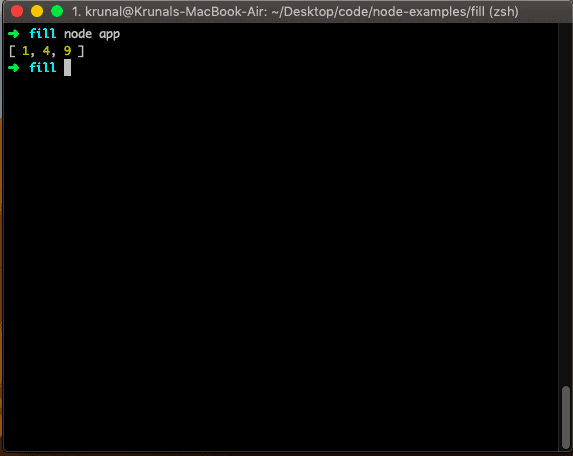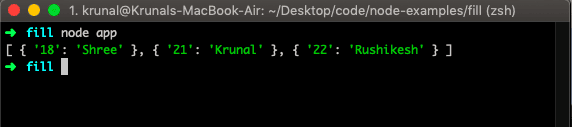# JavaScript Array map() Method

JavaScript array map() function is “used to create a new array from calling a function for every array element”. It calls the provided callback function once for each element in the array and order and constructs the new array from the results.

### Syntax

``array.map(function(currentValue, index, arr), thisValue)``

### Parameters

currentValue: It is a required parameter and the element’s current value.

index: It is an optional parameter and is the current element’s index.

arr: It is an optional parameter. The array object to the current element belongs.

Under the hood, the map function passes three arguments to your callback:

1. The current item in the array
2. The array index of the current item
3. The entire array you called map on

### Return value

It returns a new array with each element resulting from the callback function.

## Example 1: How to use the array map() method

``````let numbers = [1, 2, 3];
let squares = numbers.map(item => item * item);

console.log(squares);``````

In the above example, the numbers array will be traversed and perform the square operation to each item of an array and return a new array with those items filled. So the new array will be the squared items of each element.

Output## Example 2: How to use map() over an array of objects

We can also use the map() method to format the object in the array.

``````let gtu = [
{enrollnumber: 18, name: 'Shree'},
{enrollnumber: 21, name: 'Krunal'},
{enrollnumber: 22, name: 'Rushikesh'}
];

let mappedArrayObj = gtu.map(obj => {
let newObj = {};
newObj[obj.enrollnumber] = obj.name;
return newObj;
});

console.log(mappedArrayObj);``````

In the above example, we have taken an array of objects. The object has two properties. So we have reformated the new object by creating the first object’s value as a key and the second object’s value as a value.

Output## Example 3: Accessing an index of an array in a map() method

We can access the index by bypassing the second argument to the callback function.

``````let no = [1, 3, 4, 5];

no.map((item, i) => console.log(i));``````

Now, you can access all the indexes of the current item being iterated.

We can define the function separately, pass the value to that function, and get the result.

``````let no = [1, 3, 4, 5];

const square = (x) => {
return x*x;
}

let newArray = no.map((item, i) => square(item));

console.log(newArray);``````

In the above example, we have defined the function separately and passed the current item to that function, returning the square of that item.

The Array map() method is a pure function; it is familiar if you have any experience in Redux. It will always return a new array which is the principle of immutability.

## Example 4: Calling map() on non-array objects

The map() method reads the length property of this and then accesses each integer index.

``````const arrayLike = {
length: 3,
0: 2,
1: 3,
2: 4,
};

console.log(Array.prototype.map.call(arrayLike, (x) => x ** 2));
``````

Output

``````[ 4, 9, 16 ]
``````

## Example 5: Using map() on sparse arrays

In JavaScript, a sparse array is an array in which some elements are missing. In other words, it has gaps.

``````let sparseArray = [];

sparseArray = 'zero';
sparseArray = 'one hundred';

let newArray = sparseArray.map(x => x * 2);

console.log(newArray.length);
console.log(newArray);
``````

Output

``````101
undefined
``````

## Example 6: Mapped array contains undefined

When you use map() on an array in JavaScript, it creates a new array with the results of calling a provided function on every element in the calling array. However, if the mapping function returns undefined for an element, the new array will have undefined at that element’s position.

``````let originalArray = [1, 2, 3, 4, 5];

let newArray = originalArray.map(element => {
if (element > 3) {
return element;
}
});

console.log(newArray);
``````

Output

``````[ undefined, undefined, undefined, 4, 5 ]
``````

## Browser compatibility

 Chrome Edge Firefox Safari Opera Internet Explorer Yes Yes Yes Yes Yes 9-11

That’s it.

### 1 thought on “JavaScript Array map() Method”

1.thanks for the post